## NCERT Solutions for Class 9 Science Chapter 8 Motion

These Solutions are part of NCERT Solutions for Class 9 Science. Here we have given NCERT Solutions for Class 9 Science Chapter 8 Motion. LearnInsta.com provides you the Free PDF download of NCERT Solutions for Class 9 Science (Biology) Chapter 8 – Motion solved by Expert Teachers as per NCERT (CBSE) Book guidelines. All Chapter 8 – Motion Exercise Questions with Solutions to help you to revise complete Syllabus and Score More marks.

More Resources

NCERT TEXT BOOK QUESTIONS

IN TEXT QUESTIONS

Question 1.
An object has moved through a distance. Can it have zero displacement ? If yes, support your answer with an example. (CBSE 2015)
Yes, The displacement of the object can be zero. Let a boy completes one round of a circular track in 5 minutes. The distance travelled by the boy = circumference of the circular track. However, displacement of the boy is zero because his initial and final positions are same.

Question 2.
A farmer moves along the boundary of a square field of side 10 m in 40 s.
What will be the magnitude of displacement of the farmer at the end of 2 minutes 20 seconds ? (CBSE 2010, 2015)ABCD is a square field of side 10 m.
The farmer moves along the boundary of the field from the corner A via the corners B, C and D.
After every 40 s, the farmer is again at the corner A, so his displacement after every 40 s is zero.
At the end of 2 minutes 20 seconds = (2 x 60 + 20) = 140 s, the farmer will be at the corner C.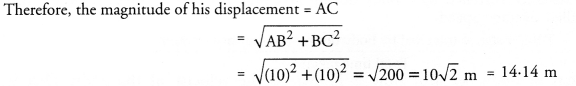Question 3.
Distinguish between speed and velocity. (CBSE 2010, 2012, 2013, 2015)

 Speed Velocity 1. Distance travelled by an object per unit time is known as its speed. The distance travelled by an object in a particular direction (i.e. displacement) per unit time is known as its velocity. 2. Average speed of a moving object cannot be zero. Average velocity of a moving object can be zero. 3. tells how fast an object moves. Velocity tells how fast an object moves and in which direction it moves. 4. Speed is a scalar quantity. Velocity is a vector quantity. 5. Speed of an object is always positive. Velocity of an object can be positive or negative.

Question 4.
Under what conditions) is the magnitude of the average velocity of an object equal to its average speed ? (CBSE 2012, 2013)
When an object moves in one direction along a straight line.

Question 5.
What does the odometer of an automobile measure ? (CBSE 2010, 2011, 2014, 2015)
Odometer of an automobile measures distance travelled by the automobile.

Question 6.
What does the path of an object look like when it is in uniform motion ? (CBSE 2012)
Straight path.

Question 7.
During an experiment, a signal from a spaceship reached the ground station in five minutes. What was the distance of the spaceship from the ground station ? The signal travels at the speed of light, that is, 3 x 108 m s-1Question 8.
When will you say a body is in

1. uniform acceleration ?
2. non-uniform acceleration (CBSE 2012, 2013)

1. A body has uniform acceleration if its velocity changes by an equal amount in equal intervals of time. .
2. A body has non-uniform acceleration if its velocity changes by unequal amount in equal intervals of time.

Question 9.
A bus decreases its speed from 80 kmh-1 to 60 kmh-1 in 5 s. Find the acceleration of the bus.
(CBSE 2010, 2011, 2012, 2015)
Here,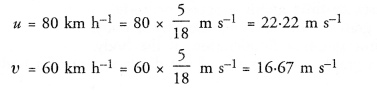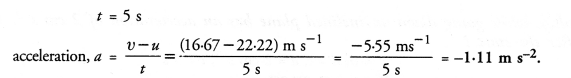Question 10.
A train starting from a railway station and moving with a uniform acceleration attains a speed of 40 km h-1 n 10 minutes. Find its acceleration.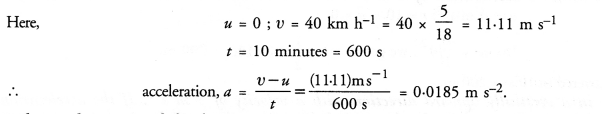Question 11.
What is the nature of the distance-time graphs for uniform and non-uniform motion of an object ?
(CBSE 2012)

1. For uniform motion, distance-time graph is a straight line having constant gradient or slope as shown in figure.
2. For non-uniform motion, distance-time graph is a curve having increasing gradient or slope or decreasing gradient as shown in figures.Question 12.
What can you say about the motion of an object whose distance-time graph is a straight line parallel to
the time axis ? (CBSE 2010, 2012)
When distance-time graph is a straight line parallel to the time axis, it means, the distance of the object is not changing with time. Thus, the object is stationary.

Question 13.
What can you say about the motion of an object if its speed-time graph is a straight line parallel to the time-axis ?
In this case, speed of the object is constant. That is, the object travels equal distances in equal intervals of time along a straight line. Hence, motion of the object is uniform motion.

Question 14.
What is the quantity which is measured by the area occupied below the velocity-time graph ?
(CBSE 2010, 2012)
Magnitude of the displacement of a body is measured by the area under the velocify-time graph.

Question 15.
A bus starting from rest moves with a uniform acceleration of 0.1 m s-2 for 2 minutes. Find
(a) the speed acquired,
(b) the distance travelled. (CBSE 2012)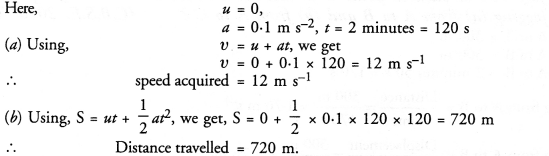Question 16.
A train is travelling at a speed of 90 km h-1. Brakes are applied so as to produce a uniform acceleration of – 0.5 m s-2 . Find how far the train will go before it is brought to rest ?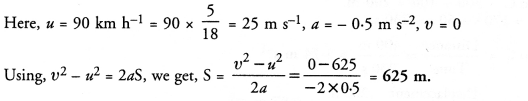Question 17.
A trolley, while going down an inclined plane has an acceleration of 2 cm s-2. What will be its velocity 3 s after the start ?
Here, u = 0,v = ?, a = 2 cm s-2, t = 3 s
Using, v = u + at, we get
v = 0 + 2 x 3 = 6 cm s-2.

Question 18.
A racing car has a uniform acceleration of 4 m s-2. What distance will it cover in 10 s after start ?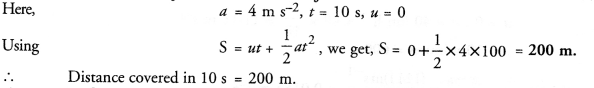Question 19.
A stone is thrown in a vertically upward direction with a velocity of 5 m s-1 If the acceleration of the stone during its motion is 10 m s-2 in the downward direction, what will be the height attained by the stone and how much time will it take to reach there ?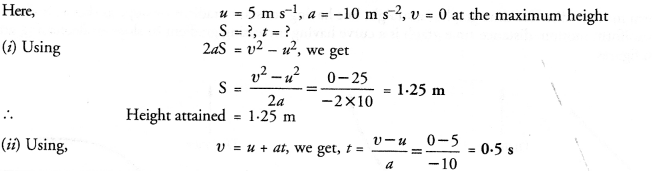NCERT CHAPTER END EXERCISE

Question 1.
An athlete completes one round of a circular track of diameter 200 m in 40 s. What will be the distance covered and the displacement at the end of 2 minutes and 20 s ?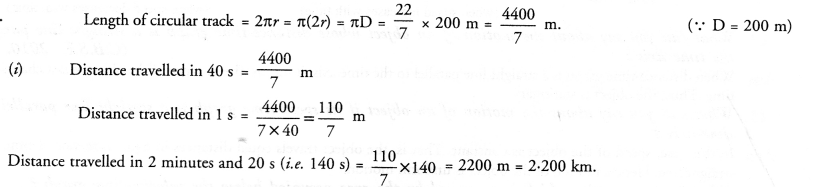(ii) After every 40 s, athlete reaches his starting point, so after 40 s, his displacement is zero. It means, the athlete completes the circular track 3 times in 120 s and in the next 20 s, he is just opposite to his starting point. Therefore, the magnitude of the displacement of the athlete at the end of the 140 s (or 2 minutes 20 s) = Diameter of the circular track = 200 m.

Question 2.
Joseph jogs from one end A to the other end B of a straight 300 m road in 2 minutes 50 seconds and then turns around and jogs 100 m back to point C in another 1 minute. What are Joseph’s average speeds and velocities in jogging
(a) from A to B and
(b) from A to C ? (CBSE 2010, Term I)
Distance from A to B – 300 m
Displacement from A to B = 300 m
Time taken to go from A to B =2 minutes 50 s = 170 s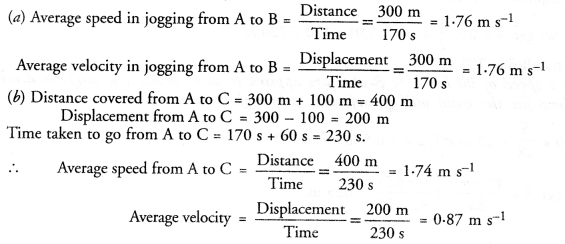Question 3.
Abdul, while driving to school, computes the average speed for his trip to be 20 km h-1. On his return trip along the same route, there is less traffic and the average speed is 40 km h-1. What is the average speed for Abdul’s trip ? (CBSE 2011)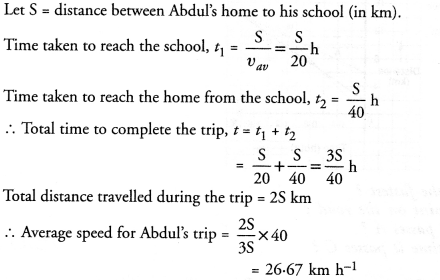Question 4.
A motor boat starting from rest on a lake accelerates in a straight line at a constant rate of 3.0 m s-2 for 8.0 s. How far does the boat travel during this time ? (CBSE 2011, 2013)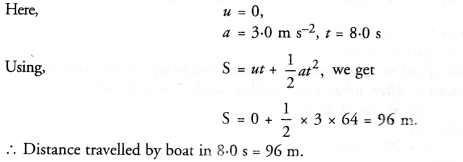Question 5.
A driver of a car travelling at 52 km h-1 applies the brakes and accelerates uniformly in the opposite direction. The car stops in 5 s. Another driver going at 3 km h-1 in another car applies his breaks slowly and stops in 10 s. On the same graph paper plot the speed versus time graphs for the two cars. Which of the two cars travelled farther after the brakes were applied ?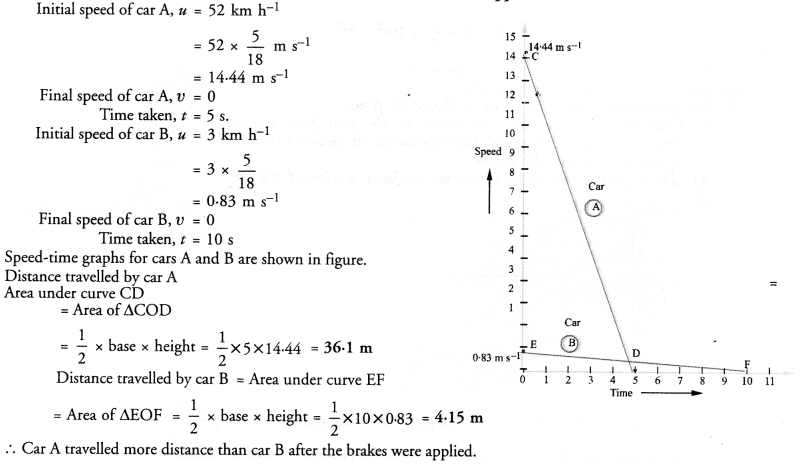Question 6.
Figure shows the distance-time graph of three objects A, B and C. Study the graph and answer the following questions :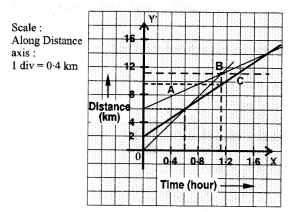(a) Which of the three is travelling the fastest ?
(b) Are all three ever at the same point on the road ?
(c) How far has C travelled when B passes A ?
(d) How far has B travelled by the time it passes C ?
(a) Speed = Slope of distance-time graph.
Since slope of distance-time graph for object B is the greatest, so object B is travelling the fastest.
(b) All the three objects will be at the same point on the road if all the three distance-time graphs intersect each other at a time. Since, all the three distance-time graphs do not intersect each other at a time, so they are never at the same point on the road.
(c) When B passes A, distance travelled by C = 9.6 – 2 = 7.6 km.
(d) Distance travelled by B by the time it passes C = 6 km.

Question 7.
A ball is gently dropped from a height of 20 m. If its velocity increases uniformly at the rate of 10 m s-2, with what velocity will it strike the ground ? After what time will it strike the ground ?Question 8.
The speed time graph for a car as shown in figure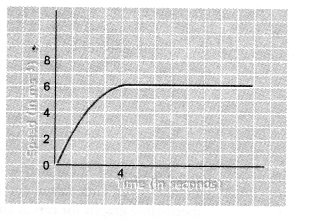(a) Find how far does the car travel in the firdt four seconds.
Shade the area on the graph that represents the distance travelled by the car during the period.
(b) Which part of the graph represents uniform motion of the car?
(a)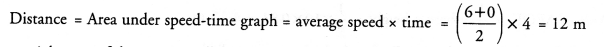(b) The straight part of the curve parallel to time axis represents the uniform motion of the car.

Question 9.
State which of the following situations are possible and give an example for each of these :
(a) an object with a constant acceleration but zero velocity.
(b) an object moving with an acceleration but with uniform speed.
(c) an object moving in a certain direction with an acceleration in the perpendicular direction.
(CBSE 2012)
(a) When an object is thrown vertically upward, its velocity at the highest point is zero but it has constant acceleration = 9.8 m s-2
(b) Uniform circular motion.
(c) An object moving in a circular path with uniform speed, its acceleration is always perpendicular to the direction of the motion of the object.

Question 10.
An artificial satellite is moving in a circular orbit of radius 42250 km. Calculate its speed if it takes 24 . hours to revolve around the earth. (CBSE 2011)